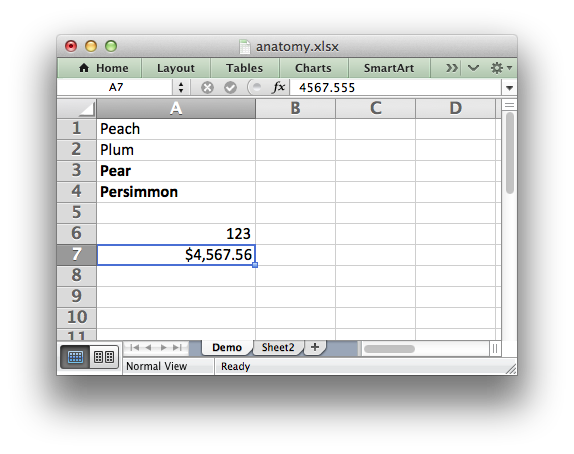libxlsxwriter
anatomy.c

Anatomy of a simple libxlsxwriter program where the program is explained line by line with comments./*
* Anatomy of a simple libxlsxwriter program.
*
* Copyright 2014-2021, John McNamara, jmcnamara@cpan.org
*
*/
#include "xlsxwriter.h"
int main() {
/* Create a new workbook. */
lxw_workbook *workbook = workbook_new("anatomy.xlsx");
/* Add a worksheet with a user defined sheet name. */
lxw_worksheet *worksheet1 = workbook_add_worksheet(workbook, "Demo");
/* Add a worksheet with Excel's default sheet name: Sheet2. */
lxw_worksheet *worksheet2 = workbook_add_worksheet(workbook, NULL);
/* Add some cell formats. */
lxw_format *myformat1 = workbook_add_format(workbook);
lxw_format *myformat2 = workbook_add_format(workbook);
/* Set the bold property for the first format. */
format_set_bold(myformat1);
/* Set a number format for the second format. */
format_set_num_format(myformat2, "\$#,##0.00");
/* Widen the first column to make the text clearer. */
worksheet_set_column(worksheet1, 0, 0, 20, NULL);
/* Write some unformatted data. */
worksheet_write_string(worksheet1, 0, 0, "Peach", NULL);
worksheet_write_string(worksheet1, 1, 0, "Plum", NULL);
/* Write formatted data. */
worksheet_write_string(worksheet1, 2, 0, "Pear", myformat1);
/* Formats can be reused. */
worksheet_write_string(worksheet1, 3, 0, "Persimmon", myformat1);
/* Write some numbers. */
worksheet_write_number(worksheet1, 5, 0, 123, NULL);
worksheet_write_number(worksheet1, 6, 0, 4567.555, myformat2);
/* Write to the second worksheet. */
worksheet_write_string(worksheet2, 0, 0, "Some text", myformat1);
/* Close the workbook, save the file and free any memory. */
lxw_error error = workbook_close(workbook);
/* Check if there was any error creating the xlsx file. */
if (error)
printf("Error in workbook_close().\n"
"Error %d = %s\n", error, lxw_strerror(error));
return error;
}
workbook_close
lxw_error workbook_close(lxw_workbook *workbook)
Close the Workbook object and write the XLSX file.
lxw_strerror
char * lxw_strerror(lxw_error error_num)
Converts a libxlsxwriter error number to a string.
workbook_new
lxw_workbook * workbook_new(const char *filename)
Create a new workbook object.
format_set_bold
void format_set_bold(lxw_format *format)
Turn on bold for the format font.
format_set_num_format
void format_set_num_format(lxw_format *format, const char *num_format)
Set the number format for a cell.
lxw_worksheet
Struct to represent an Excel worksheet.
Definition: worksheet.h:2107
lxw_error
lxw_error
Error codes from libxlsxwriter functions.
Definition: common.h:65
lxw_format
Struct to represent the formatting properties of an Excel format.
Definition: format.h:358
lxw_workbook
Struct to represent an Excel workbook.
Definition: workbook.h:280
worksheet_write_string
lxw_error worksheet_write_string(lxw_worksheet *worksheet, lxw_row_t row, lxw_col_t col, const char *string, lxw_format *format)
Write a string to a worksheet cell.
worksheet_write_number
lxw_error worksheet_write_number(lxw_worksheet *worksheet, lxw_row_t row, lxw_col_t col, double number, lxw_format *format)
Write a number to a worksheet cell.
worksheet_set_column
lxw_error worksheet_set_column(lxw_worksheet *worksheet, lxw_col_t first_col, lxw_col_t last_col, double width, lxw_format *format)
Set the properties for one or more columns of cells.